# AP Physics C: Mechanics : Understanding Orbits

## Example Questions

### Example Question #1 : Understanding Orbits

If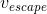is the escape velocity from the surface of a planet of massand radius. What is the velocity necessary for an object launched from the surface of a planet of the same mass, but with a radius that is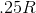, to escape the gravitational pull of this smaller, denser planet?(the same velocity for both planets)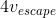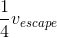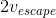Not enough informationExplanation:

Escape velocity is the velocity at which the kinetic energy of an object in motion is equal in magnitude to the gravitational potential energy. This allows us to set two equations equal to each other: the equation for kinetic energy of an object in motion and the equation for gravitational potential energy. Therefore: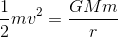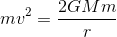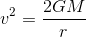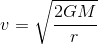In this case,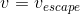. Substitute.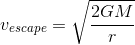Since the radius of the new planet is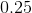orthe radius of the original planet, and they have the same masses, we can equate: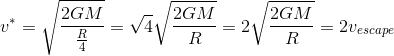Whereis the escape velocity of the larger planet and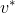is the escape velocity of the smaller planet.

### Example Question #2 : Understanding Orbits

A comet is in an elliptical orbit about the Sun, as diagrammed below: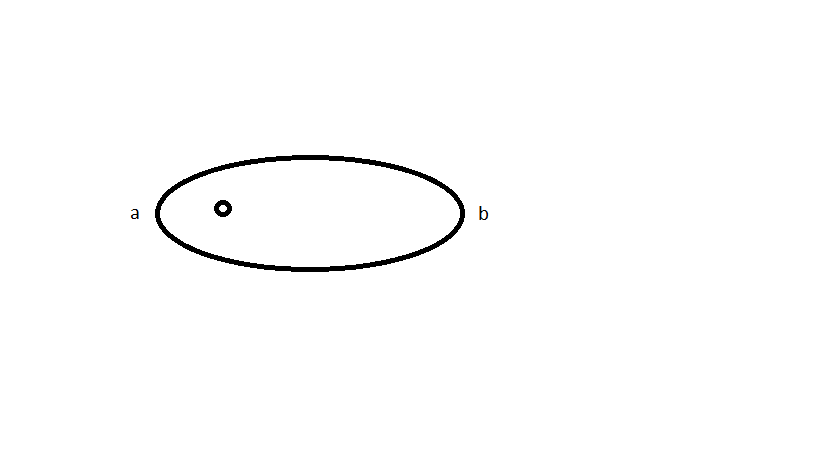The mass of the comet is very small compared to the mass of the Sun.

In the diagram above, how does the net torque on the comet due to the Sun's gravitational force compare at the two marked points?

The torque is the same at points a and b, but it is not zero

It cannot be determined from the information given

The torque is zero at both a and b

The torque is greater at point a

The torque is greater at point b

The torque is zero at both a and b

Explanation:

Since the direction of the gravitational force is directly towards the center of the Sun, it lies in the plane of the comet's orbit. Since torque is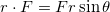, the torque is always zero. That is why the comet's angular momentum is conserved in orbit.

### Example Question #111 : Mechanics Exam

A satellite of massis in an elliptical orbit about the Earth. It's velocity at perigee is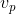and its orbital radius at perigee is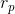. If the radius at apogee is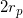, what is its velocity at apogee?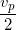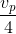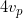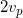Explanation:

Since the gravitational force cannot exert torque on the satellite about the Earth's center, angular momentum is conserved in this orbit: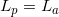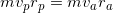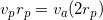### Example Question #112 : Mechanics Exam

A satellite is in an elliptical orbit about the Earth. If the satellite needs to enter a circular orbit at the apogee of the ellipse, in what direction will it need to accelerate?

Towards Earth

Away from Earth

Opposite the direction of travel

In the direction of travel

This maneuver cannot be accomplished